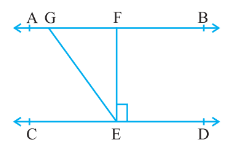Q

# In Fig. 6.30, if AB || CD, EF ⊥ CD and ∠ GED = 126°, find ∠ AGE, ∠ GEF and ∠ FGE.

3.  In Fig. 6.30, if AB  CD, EF CD and GED = 126°, find AGE, GEF and FGE.ViewsGiven AB || CD, EFCD and GED =
In the above figure,
GE is transversal. So, that AGE = GED =   [Alternate interior angles]
Also, GEF = GED - FED
=

Since AB is a straight line
Therefore, AGE  + FGE =
So, FGE =

Exams
Articles
Questions